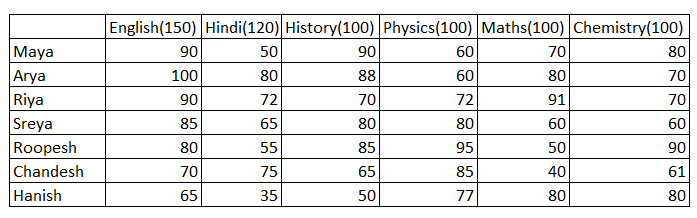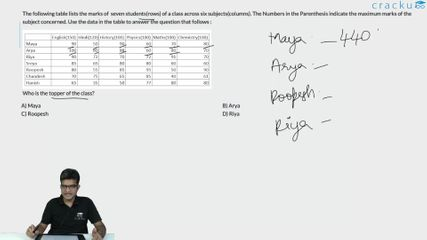Question 3

# The following table lists the marks of  seven students(rows) of a class across six subjects(columns). The Numbers in the Parenthesis indicate the maximum marks of the subject concerned. Use the data in the table to answer the question that follows :Who is the topper of the class?

Solution

The topper of the class is the one who gets a highest overall score. Let us check the options, who has got the overall score.

Option A:

The overall score of Maya = 90 + 50 + 90 + 60 + 70 + 80 = 440 marks

Option B:

The overall score of Arya = 100 + 80 + 88 + 60 + 80 + 70 = 478 marks

Option C:

The overall score of Rupesh = 80 + 55 + 85 + 95 + 50 + 90 = 455 marks

Option D:

The overall score of Riya = 90 + 72 + 70 + 72 + 91 + 70 = 465 marks

So, the topper of the class is Arya, with a overall score of 478 marks.

### View Video Solution